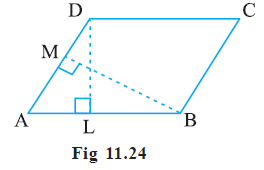# Ex.11.2 Q6 Perimeter and Area - NCERT Maths Class 7

Go back to  'Ex.11.2'

## Question

$$DL$$ and $$BM$$ are the heights on sides $$AB$$ and $$AD$$ respectively of parallelogram $$ABCD$$ (See the below figure). If the area of the parallelogram is $$1470 \,\rm cm^2$$, $$AB$$$$= 35 \,\rm cm$$ and $$AD$$ = 49 cm, find the length of $$BM$$ and $$DL$$.Video Solution
Perimeter And Area
Ex 11.2 | Question 6

## Text Solution

What is known?

The area of the parallelogram and its two sides, $$AB$$ and $$AD$$. $$DL$$ and $$BM$$ are the heights on sides $$AB$$ and $$AD$$ respectively of parallelogram $$ABCD$$.

What is unknown?

The length of the perpendiculars $$DL$$ and $$BM$$ to sides $$AB$$ and $$AD$$.

Reasoning:

$$AB \,$$is the base of the parallelogram and the perpendicular on $$AB \,$$is $$DL$$ (the height). The area of parallelogram is given as $$1470 \,\rm cm^2.$$ By using the formula of area of parallelogram we can find the height $$DL$$. Similarly, if you take $$AD$$ as the base and $$BM$$ as perpendicular (height) on $$AD$$, again by using the formula of area of parallelogram we can find the length of $$BM$$.

Steps:

Given, $$AB$$$$= 35 \,\rm cm$$, $$BC = 49 \rm \,cm$$ and Area $$= 1470 \,\rm cm^2$$

Area of parallelogram ABCD $$=$$  Base $$(AB)$$ $$\times$$ Height $$(DL)$$

\begin{align}1470 &= 35 \times {{DL}}\\{{DL}} &= \frac{{1470}}{{35}}\\{{DL}} &= 42\;\rm cm\end{align}

Area of parallelogram ABCD  $$=$$  Base $$(BC)$$ $$\times$$ Height $$(BM)$$

\begin{align}1470 &= 49 \times {{BM}}\\{{BM}} &= \frac{{1470}}{{49}}\\{{BM}} &= 30\;\rm cm\end{align}

Learn from the best math teachers and top your exams

• Live one on one classroom and doubt clearing
• Practice worksheets in and after class for conceptual clarity
• Personalized curriculum to keep up with school